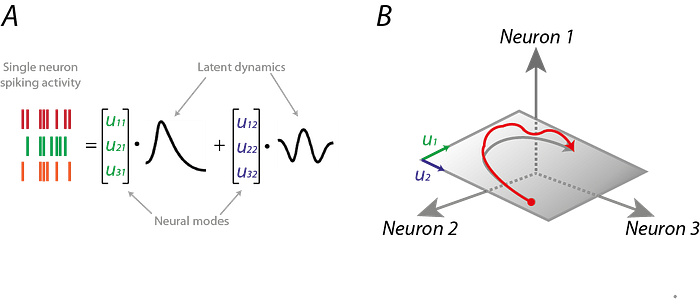# Manifold Hypothesis

The Manifold Hypothesis states that real-world high-dimensional data (images, neural activity) lie on low-dimensional manifolds manifolds embedded within the high-dimensional space. ...manifolds are topological spaces that look locally like Euclidean spaces.

The Manifold Hypothesis explains (heuristically) why machine learning techniques are able to find useful features and produce accurate predictions from datasets that have a potentially large number of dimensions ( variables). The fact that the actual data set of interest actually lives on in a space of low dimension, means that a given machine learning model only needs to learn to focus on a few key features of the dataset to make decisions. However these key features may turn out to be complicated functions of the original variables. Many of the algorithms behind machine learning techniques focus on ways to determine these (embedding) functions. What is the Manifold Hypothesis? | DeepAIManifold Learning and Dimensionality Reduction for Data Visualization... - Stefan Kühn Dimensionality Reduction methods like PCA - Principal Component Analysis - are widely used in Machine Learning for a variety of tasks. But besides the well-known standard methods there are a lot more tools available, especially in the context of Manifold Learning. We will interactively explore these tools and present applications for Data Visualization and Feature Engineering using scikit-learn.
 My understanding of the Manifold Hypothesis | Machine learning Kartik C